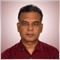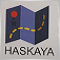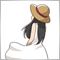83

```#property strict
#property indicator_separate_window
#include  <Custom\Function_Indicators.mqh>
double    Buffer_VolumeIntensity[];        // Assign Buffer to indicator
//+-------------------------------------------------------------------------------------+
//| Custom indicator initialization function
//+-------------------------------------------------------------------------------------+
int OnInit()
{
// Set Indicator Levels
IndicatorSetInteger(INDICATOR_LEVELS,3);
IndicatorSetInteger(INDICATOR_LEVELCOLOR,clrSilver);
IndicatorSetDouble(INDICATOR_LEVELVALUE,0, 0.25);
IndicatorSetDouble(INDICATOR_LEVELVALUE,1, 0);
IndicatorSetDouble(INDICATOR_LEVELVALUE,2,-0.25);
IndicatorSetInteger(INDICATOR_LEVELSTYLE,STYLE_DOT);
// Set Height & #Digit for accuracy of drawing of indicator values for SubWindow
IndicatorSetInteger(INDICATOR_DIGITS,Digits);
IndicatorSetInteger(INDICATOR_HEIGHT,125);
// Set indicator buffer mapping
SetIndexBuffer(0,Buffer_VolumeIntensity);
// Set indicator style, short name for label
SetIndexStyle(0,DRAW_LINE,STYLE_SOLID,1,clrGoldenrod);
string short_Name = "Volume Intensity (" + InpBB_Period + ") ";
IndicatorShortName(short_Name);
SetIndexLabel(0,"Vol Intensity (" + InpBB_Period + ")");
return(INIT_SUCCEEDED);
}
//+-------------------------------------------------------------------------------------+
//| Custom indicator iteration function
//+-------------------------------------------------------------------------------------+
int OnCalculate(const int rates_total,      // same as the bars on a chart
const int prev_calculated,  // Unchanged Bars on Chart
const datetime &time[],
const double &open[],
const double &high[],
const double &low[],
const double &close[],
const long &tick_volume[],
const long &volume[],
{
// define variables
int i; int j; int limit;
if(prev_calculated < 0)
return(-1);
if(prev_calculated == 0)
limit = (rates_total - InpBB_Period);
else
limit = (rates_total - prev_calculated + 1);
// For..Loop to calculate values and populate the buffer
for (i = limit; i >= 0; i--)
{
double sum_Intensity = 0.0;
int count = i + (InpBB_Period - 1);
int n = 0;
// High - Low could be zero when open and close is same value
// For..Loop to check Zero Divide Error
for(j = i; j <= count; j++)
{
if (high[j] - low[j] > 0)
{
sum_Intensity += ((2 * close[j]) - high[j] - low[j]) / (high[j] - low[j]);
n++;
}
}
Buffer_VolumeIntensity[i] = NormalizeDouble(sum_Intensity / n, Digits);
}
//--- return value of prev_calculated for next call
return(rates_total);
}
//+-------------------------------------------------------------------------------------+
```2735

high[j]
low[j])
Please change these expressions. for example iClose [i]
iClose(Symbol(),CallTimeFrame,i);83

Mehmet Bastem:
close[j]
high[j]
low[j])
Please change these expressions. for example iClose [i]
iClose(Symbol(),CallTimeFrame,i);

Thanks a lot Mehmet.

I have changed the code, however H4 and D1 time frame still not working. I want to calculate it for 21 period (which is my Bollinger period) too. How can i avoid changing period to 60 as suggested by you.

Regards.

```//+-------------------------------------------------------------------------------------+
//| BB Volume Intensity.mq4
//| Anil Varma | www.AnilHVarma.com
//| Indicator to show Bollinger Band Volume Intensity
//+-------------------------------------------------------------------------------------+
#property strict
#property indicator_separate_window
//#include  <Custom\Function_Indicators.mqh>
int    InpBB_Period = 60;
double Buffer_VolumeIntensity[];        // Assign Buffer to indicator

//+-------------------------------------------------------------------------------------+
//| Custom indicator initialization function
//+-------------------------------------------------------------------------------------+
int OnInit()
{
// Set Indicator Levels
IndicatorSetInteger(INDICATOR_LEVELS,3);
IndicatorSetInteger(INDICATOR_LEVELCOLOR,clrSilver);
IndicatorSetDouble(INDICATOR_LEVELVALUE,0, 0.25);
IndicatorSetDouble(INDICATOR_LEVELVALUE,1, 0);
IndicatorSetDouble(INDICATOR_LEVELVALUE,2,-0.25);
IndicatorSetInteger(INDICATOR_LEVELSTYLE,STYLE_DOT);
// Set Height & #Digit for accuracy of drawing of indicator values for SubWindow
IndicatorSetInteger(INDICATOR_DIGITS,Digits);
IndicatorSetInteger(INDICATOR_HEIGHT,125);
// Set indicator buffer mapping
SetIndexBuffer(0,Buffer_VolumeIntensity);
// Set indicator style, short name for label
SetIndexStyle(0,DRAW_LINE,STYLE_SOLID,1,clrGoldenrod);
string short_Name = "Volume Intensity (" + InpBB_Period + ") ";
IndicatorShortName(short_Name);
SetIndexLabel(0,"Vol Intensity (" + InpBB_Period + ")");
return(INIT_SUCCEEDED);
}
//+-------------------------------------------------------------------------------------+
//| Custom indicator iteration function
//+-------------------------------------------------------------------------------------+
int OnCalculate(const int rates_total,      // same as the bars on a chart
const int prev_calculated,  // Unchanged Bars on Chart
const datetime &time[],
const double &open[],
const double &high[],
const double &low[],
const double &close[],
const long &tick_volume[],
const long &volume[],
{
// define variables
int i; int j; int limit;
if(prev_calculated < 0)
return(-1);
if(prev_calculated == 0)
limit = (rates_total - InpBB_Period);
else
limit = (rates_total - prev_calculated + 1);
// For..Loop to calculate values and populate the buffer
for (i = limit; i >= 0; i--)
{
double sum_Intensity = 0.0;
int count = i + (InpBB_Period - 1);
int n = 0;
// High - Low could be zero when open and close is same value
// For..Loop to check Zero Divide Error
for(j = i; j <= count; j++)
{
double bar_Close = iClose(NULL,PERIOD_CURRENT,j);
double bar_High  = iHigh(NULL,PERIOD_CURRENT,j);
double bar_Low   = iLow(NULL,PERIOD_CURRENT,j);
if (high[j] - low[j] > 0)
{
sum_Intensity += ((2 * bar_Close) - bar_High - bar_Low) / (bar_High - bar_Low);
n++;
}
}
Buffer_VolumeIntensity[i] = NormalizeDouble(sum_Intensity / n, Digits);
}
//--- return value of prev_calculated for next call
return(rates_total);
}
//+-------------------------------------------------------------------------------------+
```893

It works on my PC with no problems, so that's probably an inherent problem with your PC.

There may be a problem with the history file. Deleting the HST file manually might help you.83

It works on my PC with no problems, so that's probably an inherent problem with your PC.

There may be a problem with the history file. Deleting the HST file manually might help you.
Thanks Nagisa ... will try that83

It works on my PC with no problems, so that's probably an inherent problem with your PC.

There may be a problem with the history file. Deleting the HST file manually might help you.

History does not seems to be cause of problem. I have updated the history data and still could not see indicator on H4 and D1 chart. Rest all charts it is working fine.25836

```      double bar_High  = iHigh(NULL,PERIOD_CURRENT,j);
double bar_Low   = iLow(NULL,PERIOD_CURRENT,j);
if (high[j] - low[j] > 0)```

You never set high/low to as-series, so your test is wrong. Replace the arrays with your two variables.2735

Anil Varma:

Thanks a lot Mehmet.

I have changed the code, however H4 and D1 time frame still not working. I want to calculate it for 21 period (which is my Bollinger period) too. How can i avoid changing period to 60 as suggested by you.

Regards.

No need for long code for this. iBands are enough

```// TF :240
double BbandH4Upper =iBands(NULL,PERIOD_H4,21,2,0,PRICE_LOW,MODE_UPPER,i);
double BbandH4Main  =iBands(NULL,PERIOD_H4,21,2,0,PRICE_LOW,MODE_MAIN,i);
double BbandH4Lower =iBands(NULL,PERIOD_H4,21,2,0,PRICE_LOW,MODE_LOWER,i);

// TF :D1
double BbandD1Upper =iBands(NULL,PERIOD_D1,21,2,0,PRICE_LOW,MODE_UPPER,i);
double BbandD1Main  =iBands(NULL,PERIOD_D1,21,2,0,PRICE_LOW,MODE_MAIN,i);
double BbandD1Lower =iBands(NULL,PERIOD_D1,21,2,0,PRICE_LOW,MODE_LOWER,i);```83

William Roeder:

You never set high/low to as-series, so your test is wrong. Replace the arrays with your two variables.

Thanks William

will try to correct this and update. got into developing EA for last week and lost control on indicator improvement.83

Mehmet Bastem:

No need for long code for this. iBands are enough

Thanks Mehmet Bastem

will try this and update. got into developing EA for last week and lost control on indicator improvement.